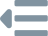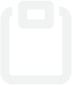iTHOR API ReferenceInstallation
Concepts
Initialization
Scenes
Object Types
Set Object States
Domain Randomization
Object Movement
Object State Changes

Move the agent in a cardinal direction, relative to it's forward facing direction. Supported action directions are

,
MoveBack
,
MoveLeft
, and
MoveRight
.controller.step(
moveMagnitude=None
)

# Other supported directions
controller.step("MoveBack")
controller.step("MoveLeft")
controller.step("MoveRight")

### Movement Parameters

Overrides the initialized step size of

gridSize
. The units are specified in meters. By default, the
gridSize
is initialized to 0.25 meters.

Changes the yaw rotation of the agent's body. Supported action directions are

RotateRight
and
RotateLeft
.controller.step(
action="RotateRight",
degrees=None
)

# Other supported direction
controller.step("RotateLeft")

### Rotate Parameters

Overrides the initialized rotation amount of

rotateStepDegrees
.

We can adjust the camera's pitch by utilizing the

LookUp
and
LookDown
commands. Both increment in $30^\circ$ intervals, with angles being clamped between $60^\circ$ in the downward direction and $30^\circ$ in the upward direction.controller.step(
action="LookUp",
degrees=30
)

# Other supported direction
controller.step("LookDown")

### Camera Rotation Parameters

Specifies the number of degrees to look up or down. Must be greater than $0$.controller.step(action="Crouch")

### Crouch Description

Crouches the agent from a standing position. The standing position is roughly $0.22$ meters taller than the crouching position.controller.step(action="Stand")

### Stand Description

Stands the agent from a crouching position. The crouching position is roughly $0.22$ meters shorter than the standing position.

Teleport
allows the agent to set its pose to anywhere in the scene in a single step. Valid poses do not place the agent outside the outer boundaries of the scene or in an area that it collides with an object.controller.step(
action="Teleport",
position=dict(x=1, y=0.9, z=-1.5),
rotation=dict(x=0, y=270, z=0),
horizon=30,
standing=True
)

### Teleport Parameters

The position of the agent's body in global 3D coordinate space. If unspecified, the position of the agent remains the same.

The rotation of the agent's body in global 3D space. Here, the rotation changes the agent's yaw rotation.

Remark

The default agent's body cannot change in pitch or roll; hence, why the $x, z=0$.

If unspecified, the rotation of the agent remains the same.

The

horizon
change's the camera's rotation. Values are clamped between [-30:60].

Since the agent looks up and down in $30^\circ$ increments, it most common for the horizon to be in $\lbrace -30, 0, 30, 60\rbrace$.

Warning

Negative camera

horizon
values correspond to agent looking up, whereas positive
horizon
values correspond to the agent looking down.

If unspecified, the horizon of the agent remains the same.

Denotes whether the agent is in a standing or crouched position. If unspecified, the standing state of the agent remains the same.

In addition to being able to

Teleport
, we also have an equivalent action
TeleportFull
that strictly requires every degree of freedom to be specified. The strictness of this action is often useful in large projects, where we don't want to any part of the agent implied implicitly.

Warning

TeleportFull
does not guarantee backwards compatibility in future releases. If a new degree of freedom is added to the agent, it will be added as a required parameter. If this is an issue, we recommend using
Teleport
.controller.step(
action="TeleportFull",
position=dict(x=1, y=0.9, z=-1.5),
rotation=dict(x=0, y=270, z=0),
horizon=30,
standing=True
)

### TeleportFull Parameters

#### position

: dict[str, float]
required

The position of the agent's body in global 3D coordinate space.

The rotation of the agent's body in global 3D space. Here, the rotation changes the agent's yaw rotation.

Remark

The default agent's body cannot change in pitch or roll; hence, why the $x, z=0$.

The

horizon
change's the camera's rotation. Values are clamped between [-30:60].

Since the agent looks up and down in $30^\circ$ increments, it most common for the horizon to be in $\lbrace -30, 0, 30, 60\rbrace$.

Warning

Negative camera

horizon
values correspond to agent looking up, whereas positive
horizon
values correspond to the agent looking down.

Denotes whether the agent is in a standing or crouched position.

It is often useful to randomize the position of the agent in the scene, before starting an episode. Here, we can use:

1. GetReachablePositions, which does an optimized BFS over a grid spaced out by the initialized
gridSize
. The valid positions are then added and returned in a list.
2. Teleport
, which can take a given position, and transform the agent to that position.

The process is illustrated below:

Step 1: Get the Positionspositions = controller.step(
action="GetReachablePositions"
).metadata["actionReturn"]
Response
[
dict(x=(...), y=(...), z=(...)),
dict(x=(...), y=(...), z=(...)),
dict(x=(...), y=(...), z=(...)),
{...}
dict(x=(...), y=(...), z=(...)),
]

Step 2: Teleport to a Positionimport random
position = random.choice(positions)
controller.step(
action="Teleport",
position=position
)

### Get Reachable Positions Response

A list of each position that the agent can be at, without colliding into any other objects or going beyond the walls.

The

Done
action nothing to the state of the environment. But, it returns a cleaned up event with respect to the metadata.controller.step(action="Done")

It is often used in the definition of a successful navigation task (see Anderson et al.), where the agent must call the done action to signal that it knows that it's done, rather than arbitrarily or biasedly guessing.

Warning

The

Done
action does literally nothing to the state of the environment. For instance, if the agent calls
Done
and then